## 스크린샷

•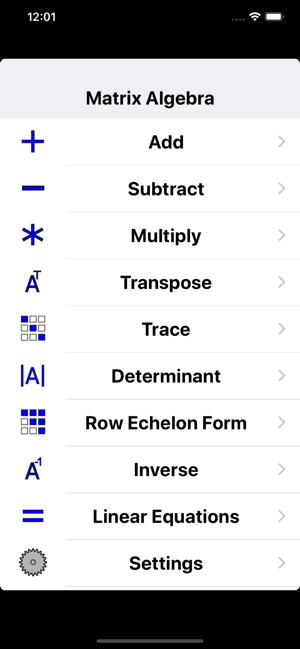•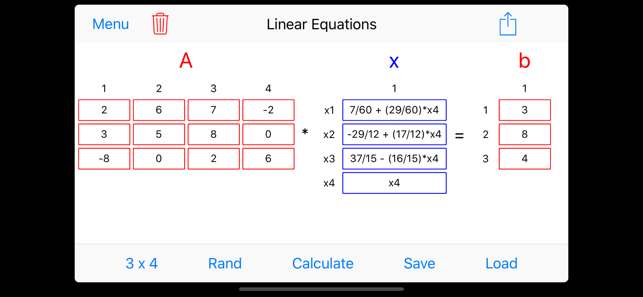•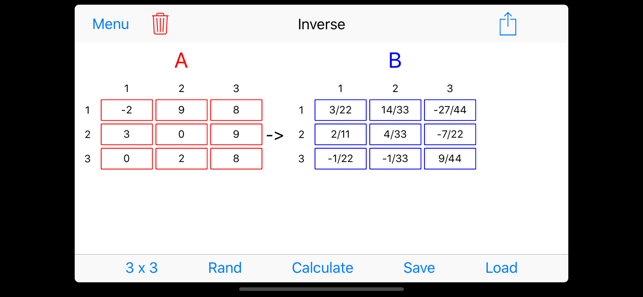•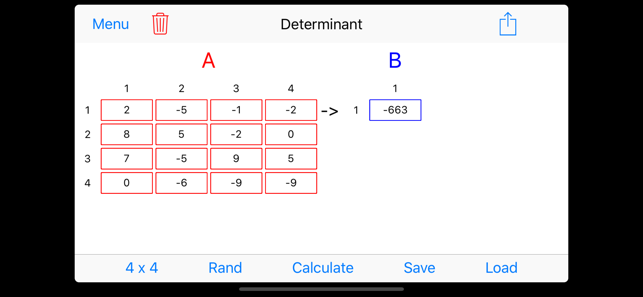•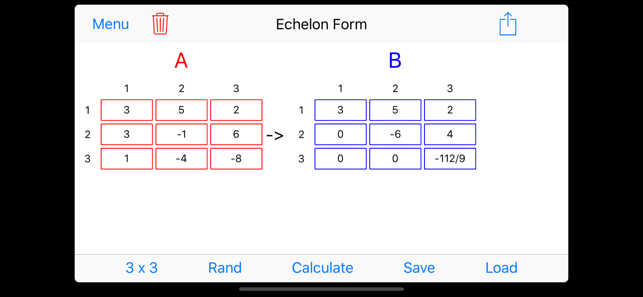## 설명

This app is suitable for use in a Linear Algebra course or any other course that involves matrix computations.

Features include:

Arithmetic:
- arbitrary precision arithmetic, able to make calculations with very large numbers
- rational arithmetic, obtain results in fractional form
- decimal calculations

Matrix Operations:
- Subtract
- Multiply
- Transpose
- Trace
- Determinant
- Gaussian Elimination
- Matrix Inverse
- Solve any system of linear equations (overdetermined, underdetermined, unique solution)

- save a matrix from one operation and load it into another, saves you from re-typing matrices
- to save a matrix, just give it a unique name, the matrix dimensions are saved as well
- delete saved matrices with a simple swipe

E-mail Saved Matrices:
- e-mail saved matrices to yourself and others
- e-mail will contain matrices in Matlab and Maple syntax so that you can directly copy and paste your matrices in Matlab or Maple

Randomized Example Problems:
- generate random practice problems by tapping the "Rand" button
- "Rand" populates the input matrices with random numbers so you can practice solving problems and check your work

## 새로운 기능

버전 1.4.0

Updated the random number generator so fractions are always in reduced form when generated.

## 정보

제공자
Remington Technologies, LLC
크기
3.4MB
카테고리
교육
호환성

iOS 8.0 버전 이상이 필요. iPhone, iPad 및 iPod touch와(과) 호환.

언어

영어

연령 등급
4+
저작권
가격
￦1,200

## 지원

•